To Vaccinate or not to Vaccinate Challenge Tutorial: How to build a machine learning model to assess sentiments
Getting started · 4 Aug 2022, 14:01

This article will provide a basic procedure on how to approach the To Vaccinate or not to Vaccinate Challenge hosted on Zindi, show you some basic techniques you can use and finally show you how to create and upload your submission to Zindi for scoring.

Use this colab notebook that has all the code used in this article to follow along and learn.

The complete description of the challenge can be found here.

### Type of Problem

This challenge aims at developing a machine learning model to assess positive, negative, or neutral Twitter posts related to vaccinations. The solution will help the governments and other public health actors monitor public sentiments towards COVID-19 vaccinations and help improve public health policy, vaccine communication strategies, and vaccination programs worldwide.

In this challenge, we are working with text data; it is initially non-numerical and must be processed before it can be fed into a Machine Learning algorithm. For this, we use Natural Language Processing - natural language processing (NLP) is the ability of a computer program to understand human language as it is spoken and written (referred to as natural language). For this challenge, we are dealing with a branch of NLP called sentiment analysis. Sentiment analysis is a field that aims to get the emotional tone behind a body of text; this is a popular way for organisations to determine and categorise opinions about a product, service, or idea.

Data understanding

Here we check the data’s quality and completeness and explore variables and their relationship.

We are provided with 4 different kinds of files:

1. variable_definitions.csv - This file describes the different variables in the data.
2. sampleSubmission.csv - This file shows the structure of your submission file.
3. train.csv - This file contains relevant data/variables that will help train the final model; It can be split into train and test to test locally.
4. CaptureSite_category.csv- This file contains the capture sites and their category.

Hint: Since this is a sentiment analysis challenge we’ll likely prioritize the textual variables.

For this challenge it seems we are working with 4 different variables:

• tweet_id: Unique identifier of the tweet
• safe_tweet: Text contained in the tweet. Some sensitive information has been removed like usernames and URLs
• label: Sentiment of the tweet (-1 for negative, 0 for neutral, 1 for positive)
• agreement: The tweets were labelled by three people. The agreement indicates the percentage of the three reviewers that agreed on the given label. You may use this column in your training, but agreement data will not be shared for the test set.

To get a clearer picture, it’s important to create a structural hypothesis with general knowledge to identify which variables are likely to affect the final output.

Data understanding is a step that gives a blueprint on what to work on in data preparation. It provides us with an idea of which variables we should focus on and transform(if need be) to work in our favour

We are going to compare how deep learning and machine learning stack to one another when it comes to solving a NLP task.

Import necessary libraries and import the required datasets:

```import io
import random
import numpy as np
import pandas as pd
import matplotlib.pyplot as plt
import re
from sklearn.model_selection import  train_test_split
# sklearn provides CountVectorizer that converts a   collection of text documents to a matrix of token counts while   TfidfVectorizer converts a collection of raw documents to a matrix of TF-IDF   features.
from sklearn.feature_extraction.text import CountVectorizer,   TfidfVectorizer
from sklearn.metrics import  mean_squared_error as mse, r2_score
from sklearn.model_selection import  KFold, StratifiedKFold
from sklearn.linear_model import  LogisticRegression

# Simpletransformers provides a simple way of training   transformers on NLP tasks
​​from simpletransformers.classification import  ClassificationModel
import warnings
warnings.filterwarnings("ignore")```

Data Exploration

Next, we explore the data and draw any important insights that might help in building a good solution through modelling.

Let's take a peek at how the training dataset looks: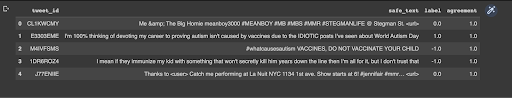We can see that we have four columns. The two most important columns for us include the safe_textcolumn and the label column. The label column is what we are trying to predict given the safe text.

Let us analyze our target variable and see whether it's balanced: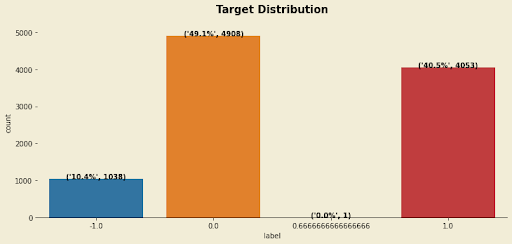From the chart above, we can see that we have some imbalance in the classes with one outlier.

This class imbalance has a significant impact on how good or powerful our solution/model will be. A good model should be able to predict all classes without any bias, i.e it should not be able to predict well just one mispredict other classes.

From the target distribution chart above we can also see that we have one outlier. To avoid confusing our model, we should drop all outliers in the target variable.

Feature engineering

According to Feature Engineering for Machine Learning by O'Reilly:

“Feature engineering is the act of extracting features from raw data and transforming them into formats that are suitable for the machine learning model. It is a crucial step in the machine learning pipeline because the right features can ease the difficulty of modelling, and therefore enable the pipeline to output results of higher quality.”

Feature engineering is an important step since it can increase the model performance and it also helps in ensuring the data input is compatible with the machine learning algorithm.

In our dataset, we have texts with hyperlinks and some funny characters as the data was scraped from Twitter. Our models are more interested in the words part of the texts. So we should do away with the hyperlinks and the funny characters. We achieve this through the code below.

```def clean_text(text):
# Remove <user>   tags
test = str(text)
text = re.sub(r'<.*?>', '',   text)
# Replace &amp; with   'and'
text = re.sub(r"&amp;", "and",   text)
# Replace punctuation   characters with spaces
filters='!"#\$%&()*+,-./:;<=>?@[\\]^_`{|}~\t\n'
translate_dict = dict((c, "   ") for c in  filters)
translate_map =   str.maketrans(translate_dict)
text = text.translate(translate_map)
# Convert text to   lowercase
text = text.strip().lower()
return  text

train.safe_text = train.safe_text.astype(str)
test.safe_text = test.safe_text.astype(str)

train['cleaned_text'] =   train.safe_text.apply(lambda x: clean_text(x))
test['cleaned_text'] = test.safe_text.apply(lambda x:   clean_text(x))```

Results of cleaning the dataset: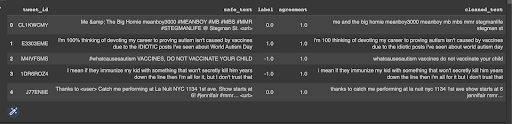Next, we can explore and see how many words we have for each tweet: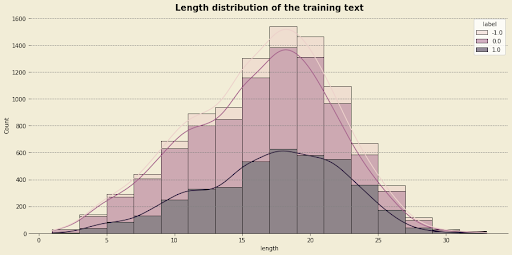From the chart above we can see that tweets which are pro-vaccination are way shorter when compared with tweets that are not for vaccination.

We can also see that the length of the tweets in the dataset follows a normal distribution. This tells us that we don't have any outliers in our dataset, which is a good thing. If there are outliers in our dataset, our models will struggle to capture real patterns when training which might result in overfitting.

Another area we can explore and get some insights which will guide us on how to build our modeling pipeline is the most frequent words used for each class.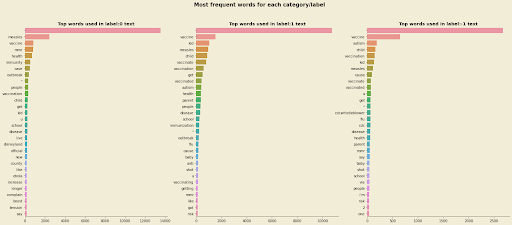The chart above shows the top 30 frequently used words for each class. One observation we can make from the chart is that the word vaccination is top of all the classes. This means that our models will need to use other patterns to differentiate between tweets that are pro-vaccination.

We can also use word clouds to see the weights/frequencies of the top words for each class.

Word cloud for tweets that are pro-vaccination: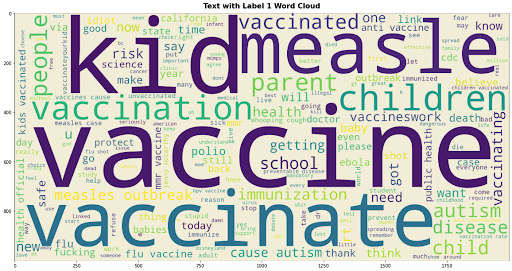Word cloud for tweets that are not for or pro-vaccination, i.e neutral tweets: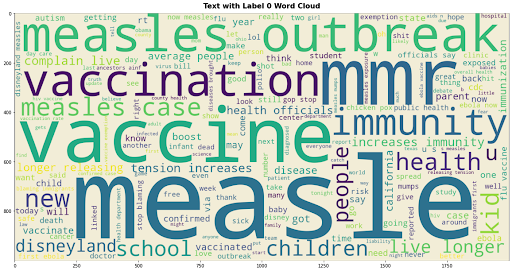When comparing the two-word clouds between neutral and pro-vaccination tweets we can see that the neutral tweets are talking more about measles vaccination and not covid vaccination.

Model training

For text data, the first step is to transform a piece of text into a canonical form. Lemmatization is an example of normalization. A lemma is the dictionary form of a word - It is the process of reducing multiple inflexions to that single dictionary form.

After the normalized text, we can take up the next step of encoding it into numerical form. The goal is to identify the particular features of the text that will be relevant to us for the specific task we want to perform - and then get these features extracted in numerical form that is accessible by the ML algorithm. This can be done by turning a piece of text into a vector.

Common approaches include:

• TfidfVectorizer
• Vectorization
• Word Embedding

The approach of TD-IDF is to give less importance to words that contain less information and are common in documents, such as ‘the’ and ‘this’ - and to give higher priority to words that have relevant information and appear less frequently. Thus TD-IDF assigns weights to terms to signify their relevance in the documents.

The first step is to split the train data into training and validation sets. Data splitting is done to avoid overfitting - meaning the data fits too well to the train data and fails reliably to fit additional data:

```#Split data into training and validation
X_train, X_valid, y_train, y_valid = train_test_split(train, train.label,   stratify=train.label, random_state=seed, test_size=0.2,shuffle=True)```

For this challenge, we will build and compare two models and choose the one with more predictive power.

Logistic regression

We are going to use the Logistic Regression and TfidfVectorizer algorithm. Logistic regression is a statistical analysis method to predict a binary outcome, such as yes or no, based on prior observations of a data set. A logistic regression model predicts a dependent data variable by analyzing the relationship between one or more existing independent variables.

```#   Always start with these features. They work (almost) everytime!
tfv = TfidfVectorizer(min_df=3,  max_features=None,
strip_accents='unicode', analyzer='word',token_pattern=r'\w{1,}',
ngram_range=(1, 3), use_idf=1,smooth_idf=1,sublinear_tf=1,
stop_words = 'english')

#   Fitting TF-IDF to both training and test sets
X_train_ctv =  tfv.fit_transform(X_train.cleaned_text)
X_valid_ctv = tfv.transform(X_valid.cleaned_text)
X_test_ctv = tfv.transform(test.cleaned_text)```
`Training the model:`
```# Stratified cross validation with 10 folds
n_folds = 10
folds = StratifiedKFold(n_splits=n_folds, shuffle=True,   random_state=seed)
total_score = 0
test_predictions_probas = []

for i, (train_indices,val_indices) in enumerate(folds.split(X_train_ctv,   y_train)):
#   Building the subsets
sub_X_train,sub_y_train =   X_train_ctv[train_indices], np.take(y_train, train_indices, axis=0)
sub_X_val,sub_y_val =   X_train_ctv[val_indices], np.take(y_train, val_indices, axis=0)

#   Training the model
model = LogisticRegression(C=1,   penalty='l2')
model.fit(sub_X_train,   sub_y_train)

#   Calculation RMSE score on validation set
val_predictions_proba =   model.predict_proba(sub_X_val)
#   Shifting the probability
val_prediction =   [(pred.argmax()-1)*pred[pred.argmax()]
for  pred in val_predictions_proba]
score = np.sqrt(mse(sub_y_val,   val_prediction))
print("Fold   {}: {}".format(i, score))
total_score += score

#   Predicting probabilities using the current model
test_prediction =   model.predict_proba(X_test_ctv)
test_predictions_probas.append(test_prediction)

print("Machine Learning Average score: {}".format(total_score/n_folds))```

Logistic regression achieves a local score of 0.59

Deep learning

We will be using the simple transformers python library to build our deep learning model.

```# Function to train model
def get_model(model_type,   model_name, n_epochs = 2, train_batch_size = 112,   eval_batch_size = 144, seq_len = 134, lr   = 1.8e-5):
model =   ClassificationModel(model_type, model_name,num_labels=1,   args={'train_batch_size':train_batch_size,"eval_batch_size":   eval_batch_size,
'reprocess_input_data': True,
'overwrite_output_dir': True, 'fp16': False,
'do_lower_case': False, 'num_train_epochs':   n_epochs,
'max_seq_length':   seq_len,'regression': True,
'manual_seed': 2, "learning_rate":lr,
"save_eval_checkpoints": False,
"save_model_every_epoch": False,})
return  model```

Then we train our model across a ten-fold cross-validation strategy:

```# Train model
total_score = 0
test_predictions_probas = []

for i, (train_indices,val_indices) in  enumerate(folds.split(train_df, train_df.labels)):
X_train, X_test =   train_df.loc[train_indices], train_df.loc[val_indices]

model = get_model('roberta', 'roberta-large',   n_epochs=3, train_batch_size=16,   eval_batch_size=16, lr = 5e-6)

model.train_model(X_train)

preds_val =   model.eval_model(X_test)
preds_val = np.clip(preds_val, -1, 1)

mse_score =   mean_squared_error(X_test['labels'], preds_val)**0.5
total_score += score

print(f"Fold   {i}: {mse_score}")

test_preds =   model.eval_model(test_df)
test_preds =   np.clip(test_preds, -1, 1)

test_predictions_probas.append(test_preds)

print("Deep Learning Average score: {}".format(total_score/n_folds))```

From the two models above, we can see that deep learning with simple transformers performs well when compared to the Logistic Regression model. This is because the deep learning transformers had already been training on millions of texts before, which gives it more predictive power.

Making a submission

Lastly, we save our data on the submission file. When submitting the final result, always ensure that it's in CSV format:

```# Create a submission file and upload to zindi for   scoring
test_prediction_proba = np.mean(test_predictions_probas, axis=0)
submission = pd.DataFrame({"tweet_id":test.tweet_id,   "label":test_prediction_proba})
submission.to_csv("submission.csv",   index=False)```

Conclusion

This model's performance can be improved by: